# 1749 Happy Valentine’s Day!

### Today’s Puzzle:

Here’s one more Valentine’s puzzle for you to do. Enjoy!### Factors of 1749:

1749 is divisible by 3 because 7 is half of 14 so any 3-digit number made from the digits 714 in any order is divisible by 3, and of course, 9 is divisible by 3.

1749 is divisible by 11 because 1 – 7 + 4 – 9 = -11, a multiple of 11.

• 1749 is a composite number.
• Prime factorization: 1749 = 3 × 11 × 53.
• 1749 has no exponents greater than 1 in its prime factorization, so √1749 cannot be simplified.
• The exponents in the prime factorization are 1, 1, and 1. Adding one to each exponent and multiplying we get (1 + 1)(1 + 1)(1 + 1) = 2 × 2 × 2 = 8. Therefore 1749 has exactly 8 factors.
• The factors of 1749 are outlined with their factor pair partners in the graphic below.### More About the Number 1749:

1749 is the hypotenuse of a Pythagorean triple:
924-1485-1749 which is 33 times (28-45-53).

1749 is the difference of two squares in FOUR different ways:
875² – 874² = 1749,
293² – 290² = 1749,
85² – 74² = 1749, and
43² – 10² = 1749.

# 1746 Love-Struck with Multiplication

### Today’s Puzzle:

If you solve this Valentine’s multiplication table puzzle, you might just become love-struck with multiplication! It’s a level 6 puzzle so you might find most of the clues to be tricky. Be sure to use logic as you place each number from 1 to 10 in both the first column and also in the top row. There is only one solution.### Factors of 1746:

• 1746 is a composite number.
• Prime factorization: 1746 = 2 × 3 × 3 × 97, which can be written 1746 = 2 × 3² × 97.
• 1746 has at least one exponent greater than 1 in its prime factorization so √1746 can be simplified. Taking the factor pair from the factor pair table below with the largest square number factor, we get √1746 = (√9)(√194) = 3√194.
• The exponents in the prime factorization are 1, 2, and 1. Adding one to each exponent and multiplying we get (1 + 1)(2 + 1)(1 + 1) = 2 × 3 × 2 = 12. Therefore 1746 has exactly 12 factors.
• The factors of 1746 are outlined with their factor pair partners in the graphic below.### More About the Number 1746:

Why is 1746 the sum of two squares? We can tell by looking at its PRIME factors:
2 is a power of 2,
3² is an even power, and
97 is a prime Pythagorean hypotenuse. (It is prime and one more than a positive multiple of 4).

At least one of its prime factors is a Pythagorean triple hypotenuse, and ALL of the rest of its non-Pythagorean-hypotenuse prime factors are either a power of 2 or combine to make a perfect square. Thus, 1746 is the sum of two squares. What are the two squares?
39² + 15² = 1746.

1746 is the hypotenuse of a Pythagorean triple:
1170-1296-1746, calculated from 2(39)(15), 39² – 15², 39² + 15².
It is also 18 times (65-72-97).

# 1745 How Thorny Is This Rose?

### Today’s Puzzle:

How thorny is this Valentine’s rose? That depends on if you use logic to solve it! It is a Level 5 puzzle, so some of the clues are meant to be tricky. Nevertheless, you can solve it! Just place all the numbers 1 to 10 in both the first column and the top row so that the given clues are the products of the numbers you write. There is only one solution.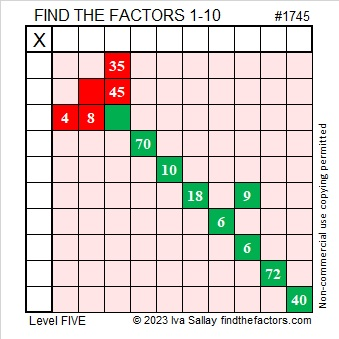Here’s the same puzzle minus the color: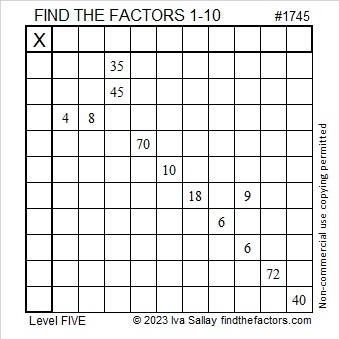### Factors of 1745:

• 1745 is a composite number.
• Prime factorization: 1745 = 5 × 349.
• 1745 has no exponents greater than 1 in its prime factorization, so √1745 cannot be simplified.
• The exponents in the prime factorization are 1 and 1. Adding one to each exponent and multiplying we get (1 + 1)(1 + 1) = 2 × 2 = 4. Therefore 1745 has exactly 4 factors.
• The factors of 1745 are outlined with their factor pair partners in the graphic below.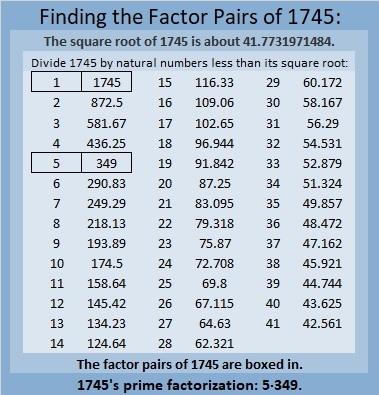### More About the Number 1745:

1745 is the difference of two squares in two different ways:
873² – 872² = 1745, and
177² – 172² = 1745.

1745 is also the sum of two squares in two different ways:
41² + 8² = 1745, and
31² + 28² = 1745.

1745 is the hypotenuse of FOUR Pythagorean triples:
177-1736-1745, calculated from 31² – 28², 2(31)(28), 31² + 28²,
656-1617-1745, calculated from 2(41)(8), 41² – 8², 41² + 8²,
900-1495-1745, which is 5 times (180-299-349)
1047-1396-1745, which is (3-4-5) times 349.

# 1744 XOXO Kisses or Multiplication? XOXO

### Today’s Puzzle:

When I was a little girl, my mother told me that OXOXOX stands for Hugs and Kisses. That would mean that O means “hug” and X means “kiss”, but I think X also means multiplication. It’s almost Valentine’s Day, so in today’s puzzle, it can mean either one.

This is a Level-4 puzzle so the clues don’t come in any particular order. Use logic to place all the numbers 1 to 10 in the first column and in the top row.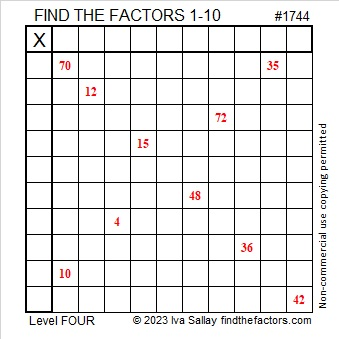### Factors of 1744:

• 1744 is a composite number.
• Prime factorization: 1744 = 2 × 2 × 2 × 2 × 109, which can be written 1744 = 2⁴ × 109.
• 1744 has at least one exponent greater than 1 in its prime factorization so √1744 can be simplified. Taking the factor pair from the factor pair table below with the largest square number factor, we get √1744 = (√16)(√109) = 4√109.
• The exponents in the prime factorization are 4 and 1. Adding one to each exponent and multiplying we get (4 + 1)(1 + 1) = 5 × 2 = 10. Therefore 1744 has exactly 10 factors.
• The factors of 1744 are outlined with their factor pair partners in the graphic below.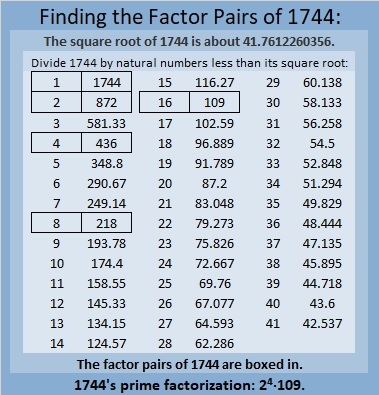### More About the Number 1744:

1744 is the difference of two squares in three ways:
437² – 435² =1744,
220² – 216² =1744, and
113² – 105² =1744.

1744 is also the sum of two squares:
40² + 12² = 1744.

1744 is the hypotenuse of a Pythagorean triple:
960-1456-1744, calculated from 2(40)(12), 40² – 12², 40² + 12².
It is also 16 times (60-91-109).

# 1743 Finding Ways to Transform My Heart

### Today’s Puzzle:

An equation of a unit circle centered at the origin is x² + y² = 1.

If we change just the “y” part of that equation, we can get a lovely heart just in time for Valentine’s Day. Try it yourself by typing the equations into Desmos.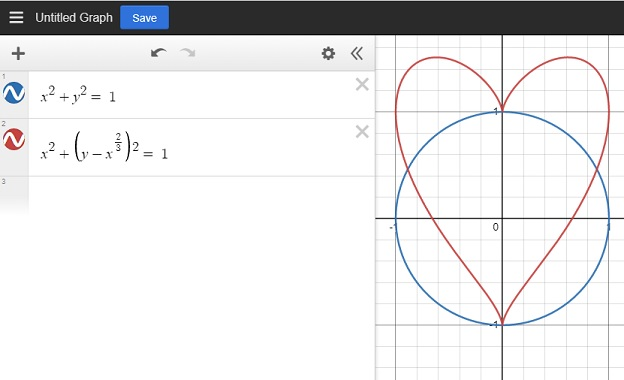There are other mathematical equations for a heart, but this is the one I’m exploring in this post.

I was puzzled over how I could transform that heart. Can I make it bigger, or dilate it? Can I slide it away from the origin or translate it? Can I rotate it? Can I reflect it across the x or y-axis?

These are questions I’d like you to explore as well.

### Heart Dilation:

In this first graphic, I was able to make my heart bigger. What kind of math let me do that? Also, how did I color the inside of some of the hearts? Look at the equations next to the heart and try to figure it out. The concentric hearts are evenly spaced. Do you recognize a pattern in the numbers that made that happen?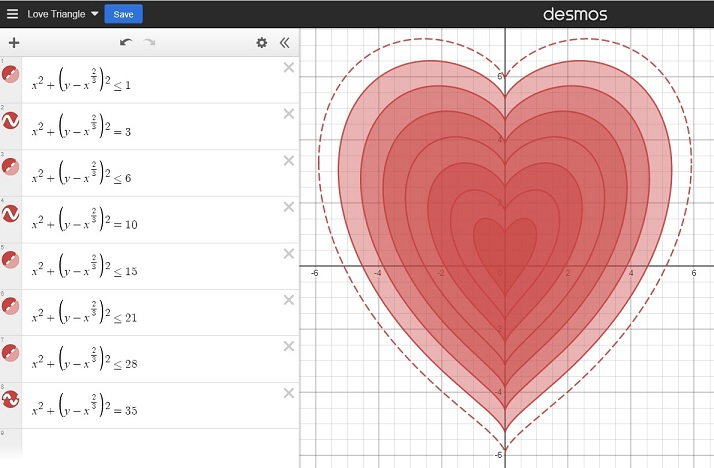### Heart Slide (Translation):

If we changed the center of a circle to (a, -b) instead of the origin, we would slide the whole circle. Here’s how we change the equation of the circle to give it a new center:
(x-a)²+(y+b)² = 1.

Similarly, in the next graphic, I was able to slide my heart away from the origin. How did I do that? Look at the equations to see how.### Heart Rotation:

A circle looks the same no matter how it is rotated, but the same isn’t true for a heart. Look at the equations below. How was I able to rotate my heart around the origin?### Heart Reflection:

Since a heart is symmetric, its reflection across the x-axis doesn’t look that interesting to me. Instead, I created a double heart that I reflected across both the x-axis and the y-axis: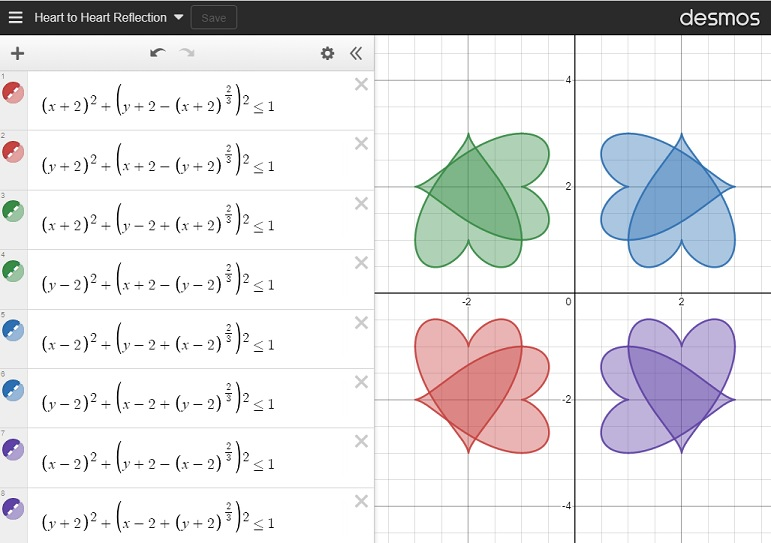### Just for Fun:

Next, I was curious about what would happen if I changed the exponents on the outside of the parenthesis, so I changed a 2 from my original equation to an 8 in a couple of different places as I moved the heart from left to right. How did changing the exponent affect my heart? I found that as long as the exponent stays even, it still looks a little like a heart.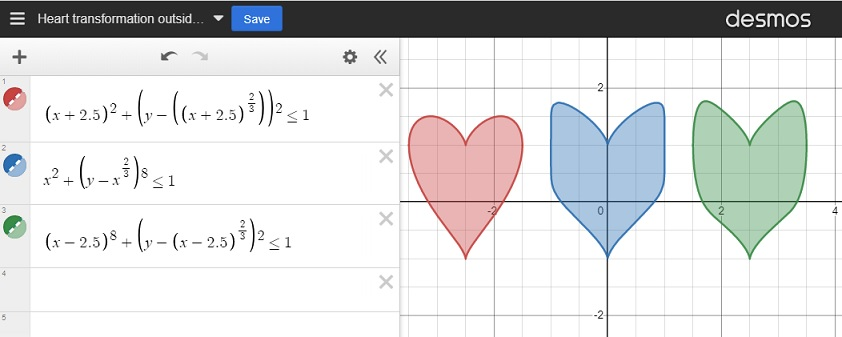I was also curious about what would happen to my heart if I changed the “2/3” to a different fraction. I used fractions less than one as well as fractions greater than one. For many of my fractions, I used the post number, 1743, as the denominator. As long as the numerator was even and the denominator was odd, the graph still looked mostly like a heart. However, the closer the fraction was to zero, the more it looked like a circle.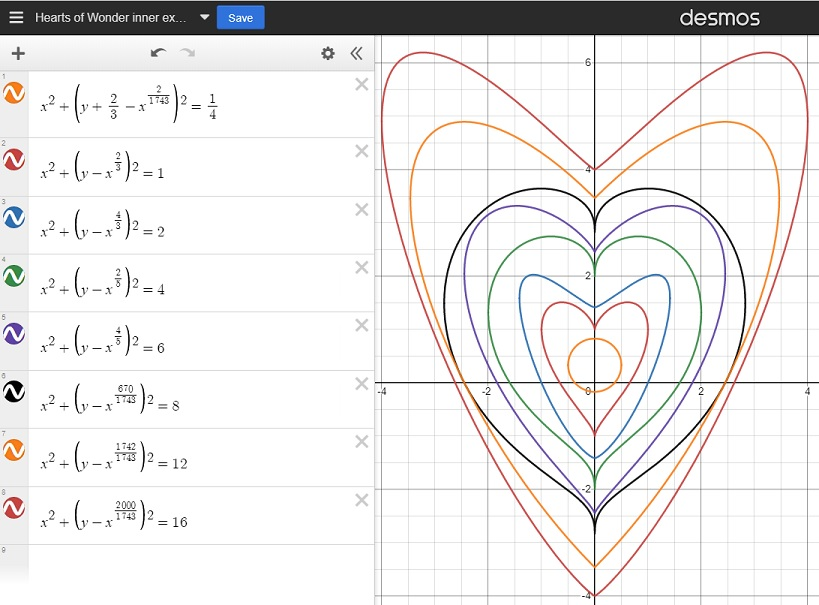Finally, I created this lovely flower using some of what I learned by making these transformations:And for just a little bit more fun, I created a simple but chaotic-looking animation that I’ve titled Hearts in Motion. Enjoy!

I had so much fun exploring this heart in Desmos. Thank you for allowing me to share my excitement with you.

### Factors of 1743:

• 1743 is a composite number.
• Prime factorization: 1743 = 3 × 7 × 83.
• 1743 has no exponents greater than 1 in its prime factorization, so √1743 cannot be simplified.
• The exponents in the prime factorization are 1, 1, and 1. Adding one to each exponent and multiplying we get (1 + 1)(1 + 1)(1 + 1) = 2 × 2 × 2 = 8. Therefore 1743 has exactly 8 factors.
• The factors of 1743 are outlined with their factor pair partners in the graphic below.### More About the Number 1743:

1743 is the difference of two squares in four different ways:
872² – 871² = 1743,
292² – 289² = 1743,
128² – 121² = 1743, and
52² – 31² = 1743.

# 1717 Factor Fits for Your Valentine

### Today’s Puzzle:

Solve this Valentine-themed Factor Fits puzzle with both logic and heart!### Factors of 1717:

• 1717 is a composite number.
• Prime factorization: 1717 = 17 × 101.
• 1717 has no exponents greater than 1 in its prime factorization, so √1717 cannot be simplified.
• The exponents in the prime factorization are 1 and 1. Adding one to each exponent and multiplying we get (1 + 1)(1 + 1) = 2 × 2 = 4. Therefore 1717 has exactly 4 factors.
• The factors of 1717 are outlined with their factor pair partners in the graphic below.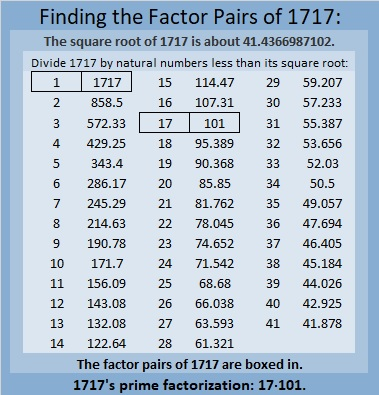### More About the Number 1717:

1717 is the sum of two squares two different ways:
41² + 6² = 1717, and
39² + 14² = 1717.

1717 is the hypotenuse of FOUR Pythagorean triples:
340 1683 1717, which is 17 times (20-99-101),
492 1645 1717, calculated from 2(41)(6), 41² – 6², 41² + 6²,
808 1515 1717, which is (8-15-17) times 101, and
1092 1325 1717, calculated from 2(39)(14), 39² – 14², 39² + 14².

# 1592 One More Valentine

### Today’s Puzzle:

I made this mystery level puzzle into one more valentine. Love can seem tricky sometimes, but I hope you enjoy working on it.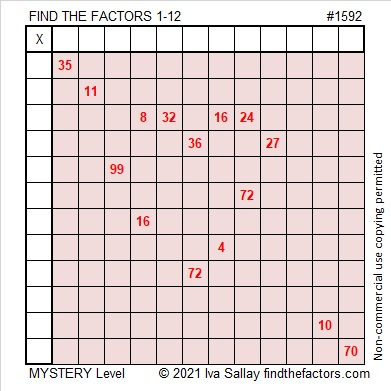### Factors of 1592:

• 1592 is a composite number.
• Prime factorization: 1592 = 2 × 2 × 2 × 199, which can be written 1592 = 2³ × 199.
• 1592 has at least one exponent greater than 1 in its prime factorization so √1592 can be simplified. Taking the factor pair from the factor pair table below with the largest square number factor, we get √1592 = (√4)(√398) = 2√398.
• The exponents in the prime factorization are 3 and 1. Adding one to each exponent and multiplying we get (3 + 1)(1 + 1) = 4 × 2 = 8. Therefore 1592 has exactly 8 factors.
• The factors of 1592 are outlined with their factor pairs in the graphic below.### More about the Number 1592:

1599 is the difference of two squares two different ways:
399² – 397² = 1592, and
201² – 197² = 1592.

# 1587 XOXOXO Hugs and Kisses OXOXOX

### Today’s Puzzle:

Hugs are often abbreviated as “O” in letters and notes, and kisses are abbreviated as “X”. This puzzle can be a Valentine’s card and will send hugs and kisses to whomever you give it.

Can you write the numbers from 1 to 12 in both the first column and the top row so that those numbers and the given clues function like a multiplication table?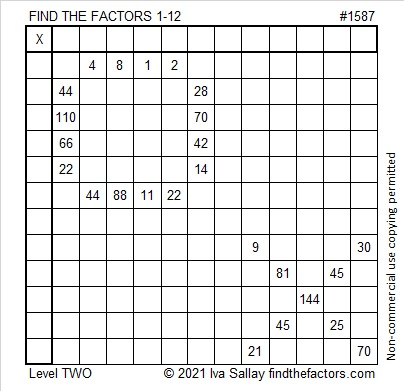### Factors of 1587:

1587 is divisible by 3 because 1 + 5 + 8 + 7 = 21, a number divisible by 3.
1587 ÷ 3 = 529. Perhaps you have memorized some perfect squares and remember that 23² = 529. (If your parents invest in a 529 college plan for your education, you are more likely to have a college degree by the time you are 23. That’s how I remember it!)

• 1587 is a composite number.
• Prime factorization: 1587 = 3 × 23 × 23, which can be written 1587 = 3 × 23².
• 1587 has at least one exponent greater than 1 in its prime factorization so √1587 can be simplified. Taking the factor pair from the factor pair table below with the largest square number factor, we get √1587 = (√529)(√3) = 23√3.
• The exponents in the prime factorization are 1 and 2. Adding one to each exponent and multiplying we get (1 + 1)(2 + 1) = 2 × 3 = 6. Therefore 1587 has exactly 6 factors.
• The factors of 1587 are outlined with their factor pair partners in the graphic below.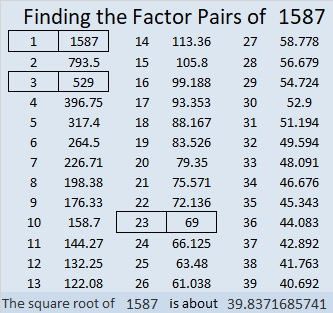### More about the Number 1587:

1587 is the difference of two squares three different ways:
794² – 793² = 1587,
266² – 263² = 1587, and
46² – 23² = 1587.